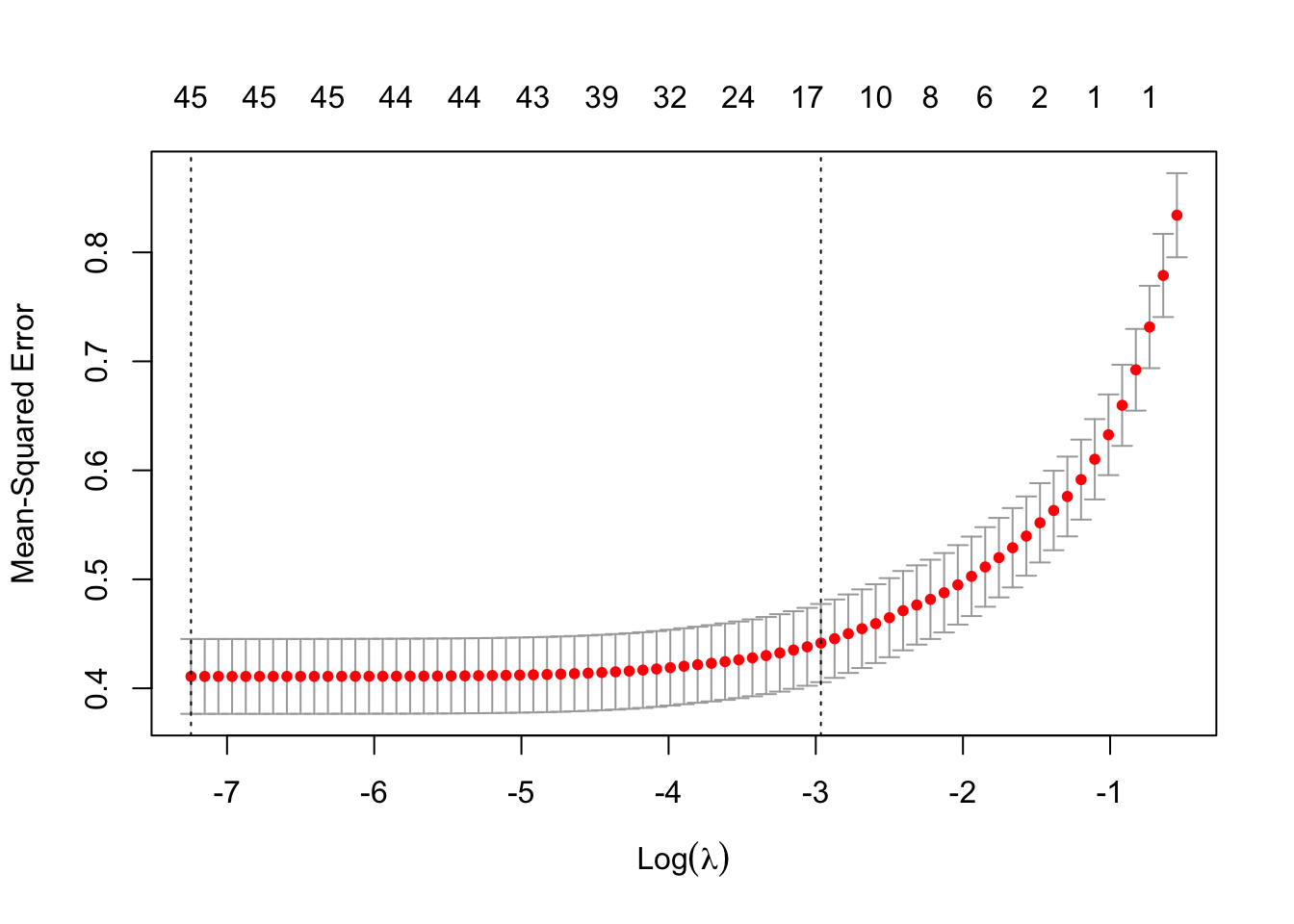This homework is going to examine the use of a) stepwise regression and b) LASSO for model selection with AIC and cross-validation. We will be re-using the home sale data we’ve saw in HW2.

# 1 Data

Load in the data. Do the same things we did last week to clean it a bit.

homes = read_csv("https://codowd.com/bigdata/hw/hw2/homes2004.csv")

#Make a function that tells us if there are two levels -- Y and N
temp_fn = function(x) is.character(x) & all(levels(as.factor(x)) %in% c("Y","N"))
# Make a function that takes those levels and makes a logical variable
temp_fn2 = function(x) x == "Y"
#Run temp fn2 on columns where temp_fn 1 is true
homes = homes %>% mutate(across(where(temp_fn),temp_fn2))
#Convert remaining character functions to
homes = homes %>% mutate(across(where(is.character),as.factor))
# But we want to relevel them for LASSO
na_relevel = function(x) factor(x,levels=c(NA,levels(x)),exclude=NULL)
homes = homes %>% mutate(across(where(is.factor),na_relevel))

Make a binary for if the number of bedrooms and bathrooms are both at least two. This is what we will be trying to predict.

homes = homes %>% mutate(twotwo = BATHS>=2 & BEDRMS >=2)

# 2 Questions

All questions asking you to predict twotwo in the data want you to use all variables. All questions not regarding twotwo should include twotwo in the model selection process (e.g. just don’t try to exclude it).

## 2.1 Q1

Model 1: Use a forward stepwise linear regression (with AIC based steps) to predict log(LPRICE) in the data. What is the AIC of the final regression (hint: its part of the standard output for glm but not lm)? How many coefficients does it have?

null = glm(log(LPRICE)~1,data=homes)
full = formula(glm(log(LPRICE)~.,data=homes))
stepwise = step(null,full,dir="forward",trace=0)
summary(stepwise)$aic #Prints AIC ##  30290.06 length(coef(stepwise)) #Prints number of coefficients ##  41 Model 2: Use a forward stepwise linear regression (with BIC based steps - hint: look at the help page for step) to predict log(LPRICE) in the data. How many coefficients does this model have? stepwise_bic = step(null,full,dir="forward",trace=0,k=log(nrow(homes))) length(coef(stepwise_bic)) ##  32 In two sentences or less, explain the reason for the difference in number of coefficients between models 1&2. BIC imposes a stronger penalty on additional parameters, multiplying each additional parameter by ~10 (log(nrow(homes)) in this data) rather than 2. This means fewer parameters are sufficiently predictive to overcome the penalty, and so fewer variables get included. ## 2.2 Q2 xmatrix = sparse.model.matrix(log(LPRICE)~.,data=homes) Use a cross-validated LASSO to predict log(LPRICE) (cv.glmnet should make this relatively easy, but you will want xmatrix from above). Show the deviance plot. How many possible subsets of the variables in our data are there? (hint: ncol(xmatrix) $$\neq$$ ncol(homes), and ncol(xmatrix) is the relevant number) Model 3: Find the $$\lambda$$ that minimizes OOS deviance and the deviance at that $$\lambda$$ (no need to report either). What is the number of non-zero coefficients with that $$\lambda_{min}$$? Model 4: Find the biggest $$\lambda$$ within 1 standard error of the minimum OOS deviance and the deviance at that $$\lambda$$ (no need to report either). How many non-zero coefficients with that $$\lambda_{1se}$$? We know that relative to the null distribution, the pseudo $$R^2$$ is $$1-D/D_0$$. What we haven’t discussed is that we could use a similar method to compare any two models (with the same likelihoods). What is the ratio of deviance of the larger model to deviance of the smaller model? In two sentences or less interpret that ratio. cv.mod = cv.glmnet(xmatrix,log(homes$LPRICE)) #Build model
2^ncol(xmatrix) #Find n subsets. 
##  1.1259e+15
plot(cv.mod)cv.mod$lambda.min #Minimizing Lambda ##  0.0007139252 min(cv.mod$cvm) #Min OOS deviance. Could also read off plot. 
##  0.4108812
sum(coef(cv.mod,s="lambda.min")!=0) #nonzero coefs with minimizing lambda.
##  46

Mostly the same code for the second bits

cv.mod$lambda.1se #1se Lambda ##  0.05155117 cv.mod$cvm[cv.mod$lambda == cv.mod$lambda.1se] #deviance of 1se Lambda
##  0.441502
sum(coef(cv.mod,s="lambda.1se")!=0) #nonzero coefs with 1se lambda
##  18
cv.mod$cvm[cv.mod$lambda == cv.mod$lambda.1se]/min(cv.mod$cvm)
##  1.074525

This ratio says that relative to the larger model, the smaller model fails to explain ~6% of the variation in the data.

## 2.3 Q3

Model 5: Add interactions (warning: may be slow, hint: you’ll need to modify/recreate xmatrix) and re-estimate the same cross-validation model as in Q2. How many possible subsets are there now (or at least, what does R say)? Show the CV error plot. What is the estimated number of coefficients when $$\lambda=0.2$$? (hint: look at the help page for cv.glmnet) In one sentence, how do these OOS deviances compare to the OOS deviances in Q2?

xmatrix =  sparse.model.matrix(log(LPRICE)~.^2,data=homes)
cv.mod2 = cv.glmnet(xmatrix,log(homes\$LPRICE))
2^ncol(xmatrix)
##  Inf
plot(cv.mod2)